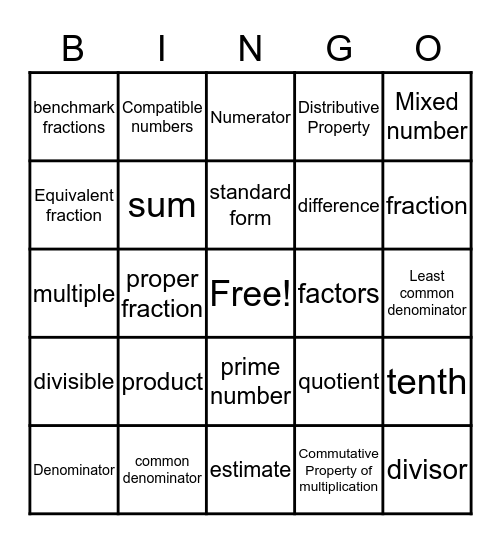# Fraction VocabularyThis bingo card has a free space and 29 words: Denominator, least common multiple, proper fraction, Identity Property of Multiplication, benchmark fractions, Numerator, sum, product, factors, common denominator, Least common denominator, divisor, estimate, Commutative Property of multiplication, fraction, dividend, prime number, divisible, Mixed number, multiple, quotient, improper fraction, difference, Equivalent fraction, Distributive Property, Compatible numbers, tenth, standard form and Whole numbers.

## Play Online